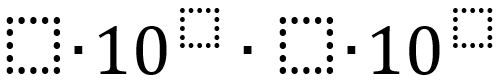# Scientific Notation

Directions: Using the digits 1 to 9 at most one time each, fill in the boxes to make the greatest (or least) product.### Hint

Where is the best place to put the biggest numbers?

Why should they go there?

7*10^9 * 6*10^8

Source: Bryan Meyer

## Square Root Expression 2

Directions: Using the digits 0 to 9 at most one time each, place a digit …

1.Smallest value?

(4*10^1)*(3*10^2)

2.how do I get this to equal 800,000,000

3.2 x 10^1 x 3 x 10^0

•digits 1-9 a 0 would not be allowed
Answer is: 4 times 10^1 times 3 times 10^2

•This set up says 1-9 only, but you could definitely allow 0-9 to see which students remember properties of zero.

But then I would use 5 x 10^ 6 X 0 x 10^9 to get the smallest value.